# ggplot2 - Introduction to Aesthetics

### Introduction

This is the fourth post in the series Elegant Data Visualization with ggplot2. In the previous post, we learnt about geoms and how we can use them to build different plots. In this post, we will focus on the aesthetics i.e.  color, shape, size, alpha, line type, line width etc. We can map these to variables or specify values for them. If we want to map the above to variables, we have to specify them within the `aes()` function. We will look at both methods in the following sections.

Explore aesthetics such as

• color
• shape
• size
• fill
• alpha
• width

### Libraries, Code & Data

We will use the following libraries in this post:

All the data sets used in this post can be found here and code can be downloaded from here.

### Data

#### Introduction

``````ecom <- readr::read_csv('https://raw.githubusercontent.com/rsquaredacademy/datasets/master/web.csv')
ecom``````
``````## # A tibble: 1,000 x 11
##       id referrer device bouncers n_visit n_pages duration country purchase
##    <dbl> <chr>    <chr>  <lgl>      <dbl>   <dbl>    <dbl> <chr>   <lgl>
##  1     1 google   laptop TRUE          10       1      693 Czech ~ FALSE
##  2     2 yahoo    tablet TRUE           9       1      459 Yemen   FALSE
##  3     3 direct   laptop TRUE           0       1      996 Brazil  FALSE
##  4     4 bing     tablet FALSE          3      18      468 China   TRUE
##  5     5 yahoo    mobile TRUE           9       1      955 Poland  FALSE
##  6     6 yahoo    laptop FALSE          5       5      135 South ~ FALSE
##  7     7 yahoo    mobile TRUE          10       1       75 Bangla~ FALSE
##  8     8 direct   mobile TRUE          10       1      908 Indone~ FALSE
##  9     9 bing     mobile FALSE          3      19      209 Nether~ FALSE
## 10    10 google   mobile TRUE           6       1      208 Czech ~ FALSE
## # ... with 990 more rows, and 2 more variables: order_items <dbl>,
## #   order_value <dbl>``````

#### Data Dictionary

• id: row id
• referrer: referrer website/search engine
• os: operating system
• browser: browser
• device: device used to visit the website
• n_pages: number of pages visited
• duration: time spent on the website (in seconds)
• repeat: frequency of visits
• country: country of origin
• purchase: whether visitor purchased
• order_value: order value of visitor (in dollars)

#### Color

In ggplot2, when we mention `color` or `colour`, it usually refers to the color of the geoms. The `fill` argument is used to specify the color of the shapes in certain cases. In this first section, we will see how we can specify the color for the different geoms we learnt in the previous post.

#### Point

For points, the `color` argument specifies the color of the point for certain shapes and border for others. The `fill` argument is used to specify the background for some shapes and will not work with other shapes. Let us look at an example:

``````ggplot(mtcars, aes(x = disp, y = mpg, color = factor(cyl))) +
geom_point()``````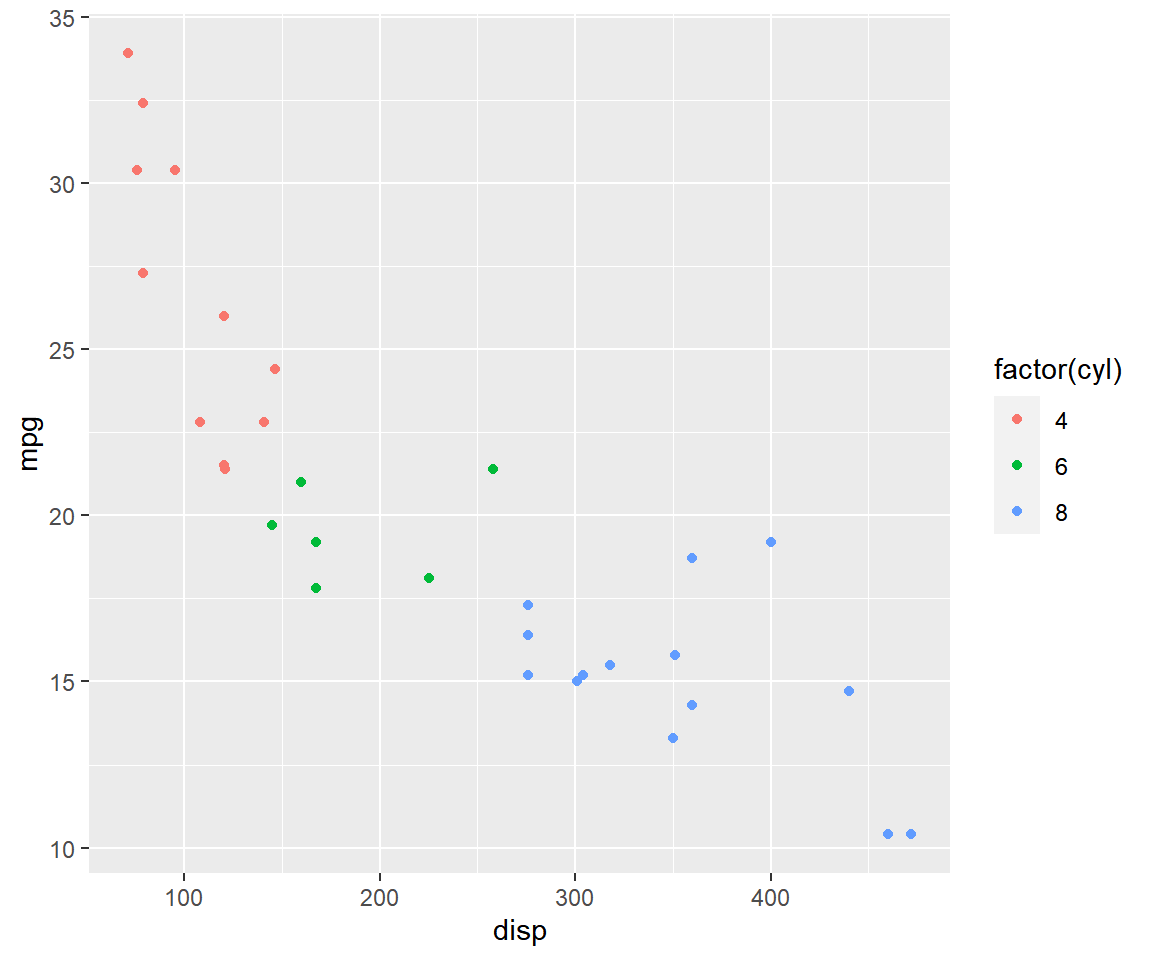We can map the variable to color in the `geom_point()` function as well since it inherits the data from the `ggplot()` function.

``````ggplot(mtcars, aes(x = disp, y = mpg)) +
geom_point(aes(color = factor(cyl)))``````If you do not want to map a variable to color, you can specify it separately using the `color` argument but in this case it should be outside the `aes()` function.

``````ggplot(mtcars, aes(x = disp, y = mpg)) +
geom_point(color = 'blue')``````Now we will change the shape of the points to understand the difference between `color` and `fill` arguments. It can be again mapped to variables or values. Let us map shape to variables.

``````ggplot(mtcars, aes(x = disp, y = mpg, shape = factor(cyl))) +
geom_point()``````Let us map shape to `cyl` in the `geom_point()` function. Remember, when you are mapping an aesthetic to a variable, it must be inside `aes()`.

``````ggplot(mtcars, aes(x = disp, y = mpg)) +
geom_point(aes(shape = factor(cyl)))``````Instead of mapping shape to a variable, let us specify a value for shape. In this case, `shape` is not wrapped inside `aes()` as we are not mapping it to a variable.

``````ggplot(mtcars, aes(x = disp, y = mpg)) +
geom_point(shape = 5)``````Let us specify a color for the point using `color` argument.

``````ggplot(mtcars, aes(x = disp, y = mpg)) +
geom_point(shape = 5, color = 'blue')``````Background color cannot be added for all shapes. In the below example, we try to modify the background color using the `fill` argument but it does not work.

``````ggplot(mtcars, aes(x = disp, y = mpg)) +
geom_point(shape = 5, fill = 'blue')``````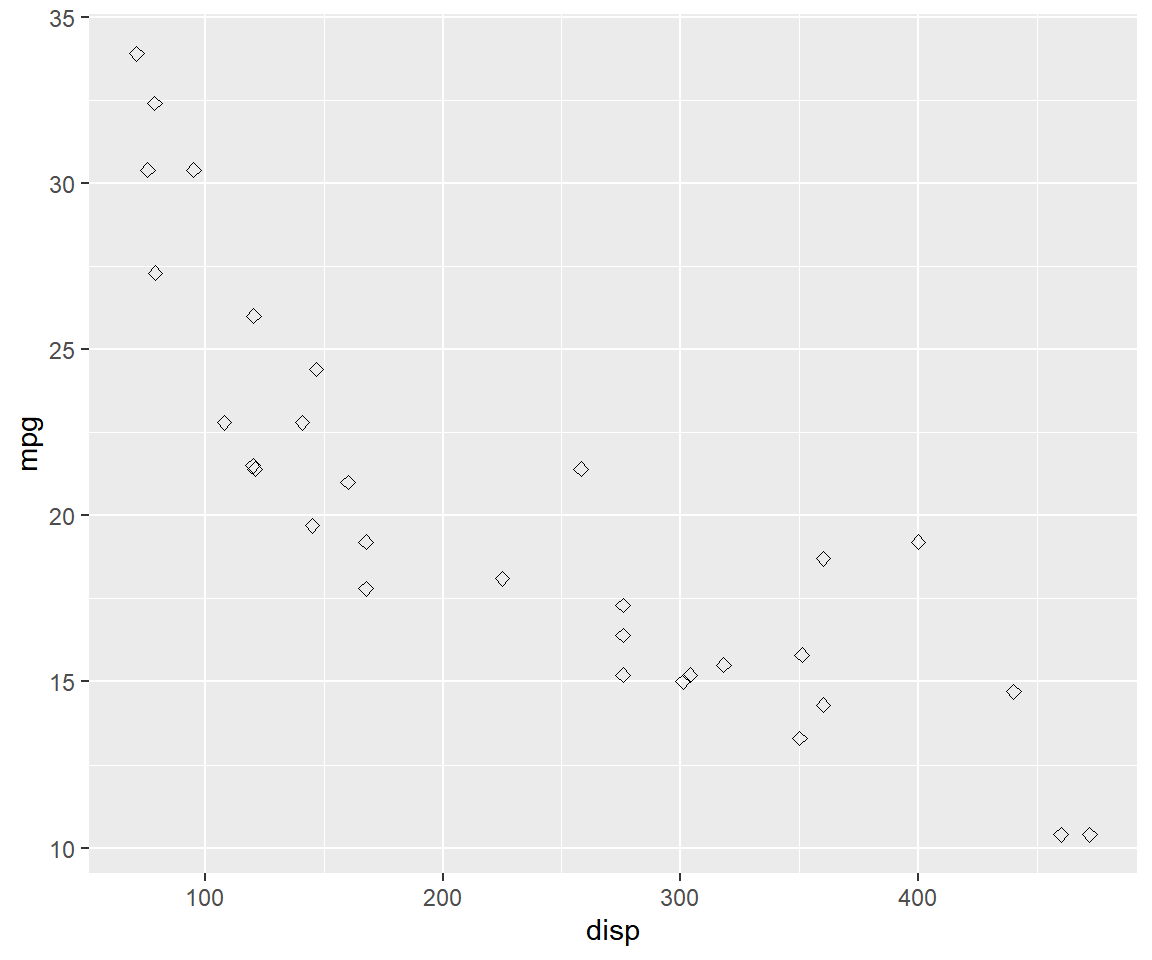Since the shape number is now greater than 21, `fill` argument will add background color in the below case.

``````ggplot(mtcars, aes(x = disp, y = mpg)) +
geom_point(shape = 22, fill = 'blue')``````In shapes greater than number 21, `color` argument will modify the border of the shape.

``````ggplot(mtcars, aes(x = disp, y = mpg)) +
geom_point(shape = 22, color = 'blue')``````Let us map size of points to a variable. It is advised to map size only to continuous variables and not categorical variables.

``````ggplot(mtcars, aes(x = disp, y = mpg, size = disp)) +
geom_point()``````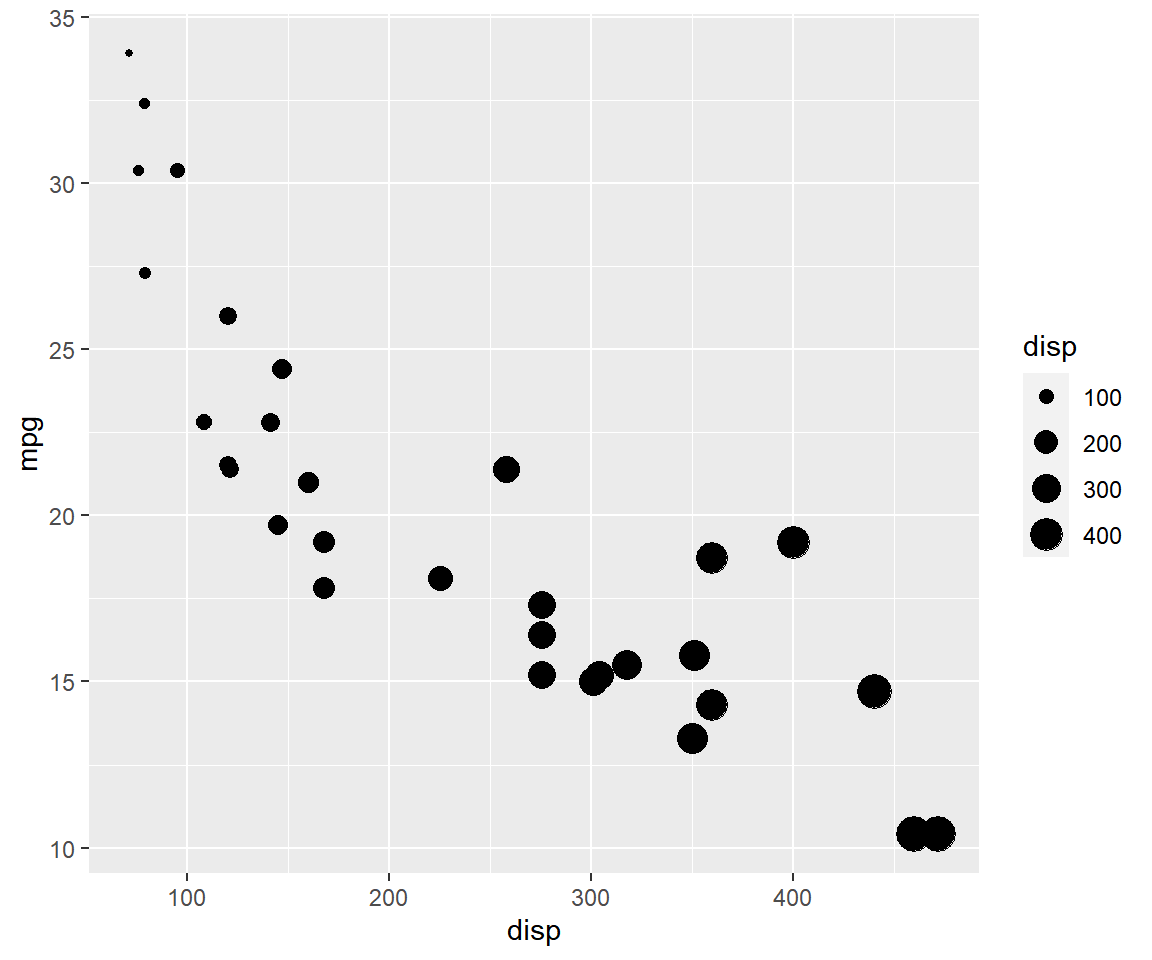If you map size to categorical variables, ggplot2 will throw a warning.

Specify value for size.

``````ggplot(mtcars, aes(x = disp, y = mpg)) +
geom_point(size = 4)``````To modify the opacity of the color, use the `alpha` argument.

``````ggplot(mtcars, aes(x = disp, y = mpg)) +
geom_point(aes(alpha = factor(cyl)), color = 'blue')``````
``## Warning: Using alpha for a discrete variable is not advised.``So far we have focussed on `geom_point()` to learn how to map aesthetics to variables. To explore line type and line width, we will use `geom_line()`. In the previous post, we used `geom_line()` to build line charts. Now we will modify the appearance of the line. In the section below, we will specify values for color, line type and width. In the next section, we will map the same to variables in the data. We will use a new data set. You can download it from here. It contains GDP (Gross Domestic Product) growth data for the BRICS (Brazil, Russia, India, China, South Africa) for the years 2000 to 2005.

### Data

``gdp <- readr::read_csv('https://raw.githubusercontent.com/rsquaredacademy/datasets/master/gdp.csv')``
``## Warning: Missing column names filled in: 'X1' ``

A line chart can be created using `geom_line()`. In the below example, we examine the GDP trend of India and modify the color of the line to `'blue'`.

``````ggplot(gdp, aes(year, india)) +
geom_line(color = 'blue')``````To modify the line type, use the `linetype` argument. It can take values between 1 and 5.

``````ggplot(gdp, aes(year, india)) +
geom_line(linetype = 2)``````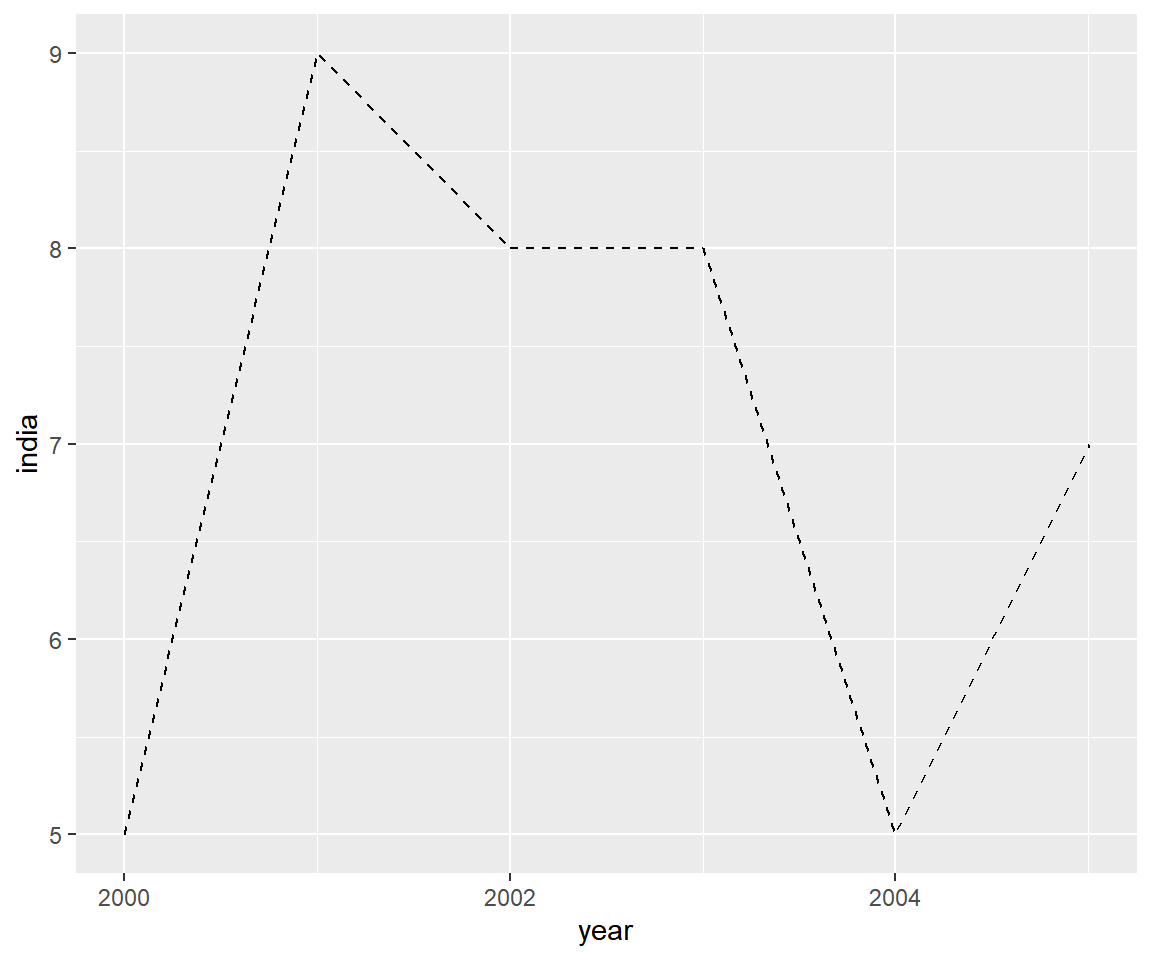The line type can also be mentioned in the following way:

``````ggplot(gdp, aes(year, india)) +
geom_line(linetype = 'dashed')``````The width of the line can be modified using the `size` argument.

``````ggplot(gdp, aes(year, india)) +
geom_line(size = 2)``````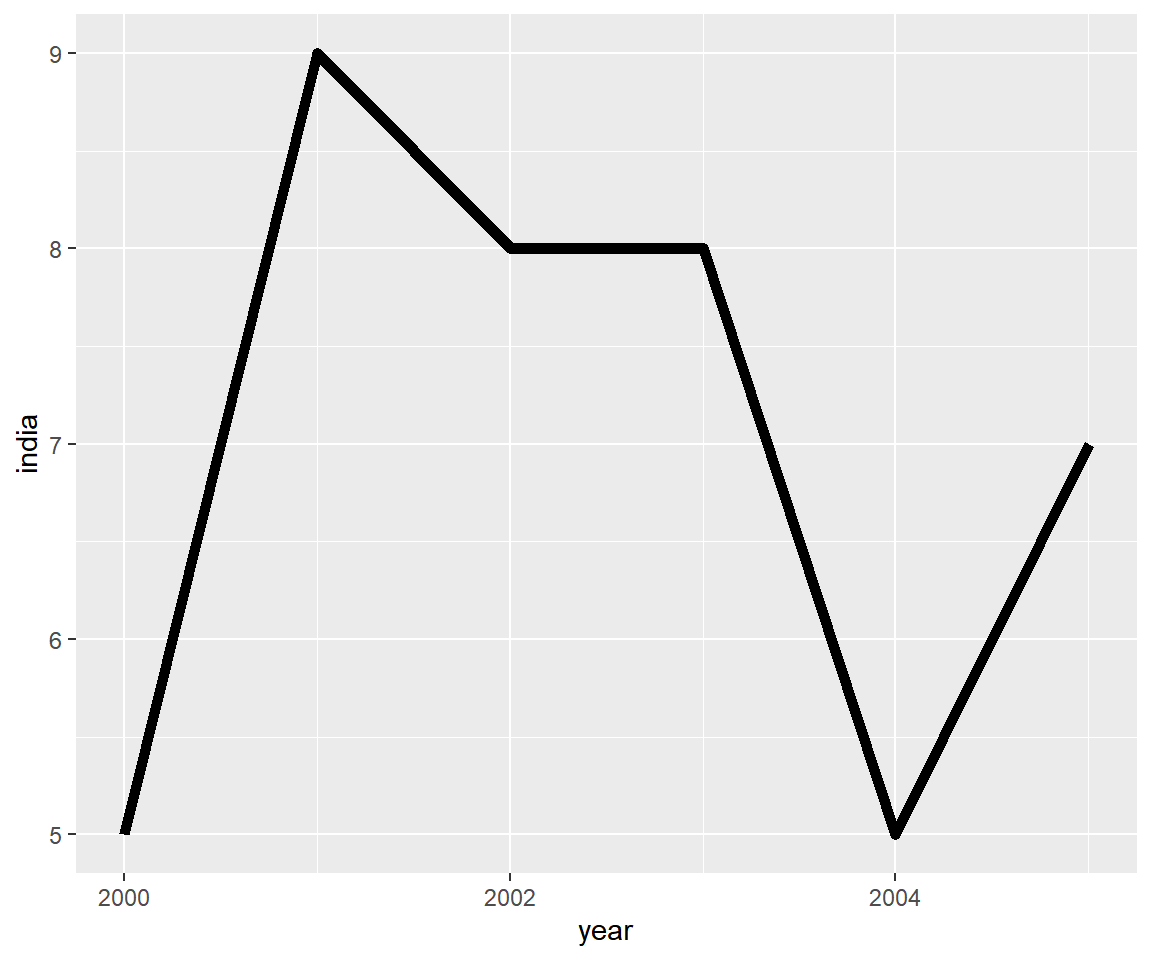Now let us map the aesthetics to the variables. The data used in the above example cannot be used as we need a variable with country names. We will use `gather()` function from the `tidyr` package to reshape the data.

``````gdp2 <-
gdp %>%
select(year, growth, india, china) %>%
gather(key = country, value = gdp, -year)

gdp2``````
``````## # A tibble: 18 x 3
##    year       country   gdp
##    <date>     <chr>   <dbl>
##  1 2000-01-01 growth      6
##  2 2001-01-01 growth      9
##  3 2002-01-01 growth      8
##  4 2003-01-01 growth      9
##  5 2004-01-01 growth      9
##  6 2005-01-01 growth      8
##  7 2000-01-01 india       5
##  8 2001-01-01 india       9
##  9 2002-01-01 india       8
## 10 2003-01-01 india       8
## 11 2004-01-01 india       5
## 12 2005-01-01 india       7
## 13 2000-01-01 china       8
## 14 2001-01-01 china       5
## 15 2002-01-01 china       6
## 16 2003-01-01 china       8
## 17 2004-01-01 china       9
## 18 2005-01-01 china       8``````

To map the aesthetics to a variable, we must use the `group` argument. In the below example, we map the aesthetics to `country`. But we cannot distinguish between the lines as their color, width and line type are the same. We have easily plotted the GDP trend of all countries using the `group` argument. Now, let us ensure that we can distinguish and identidy them using different aesthetics.

``````ggplot(gdp2, aes(year, gdp, group = country)) +
geom_line()``````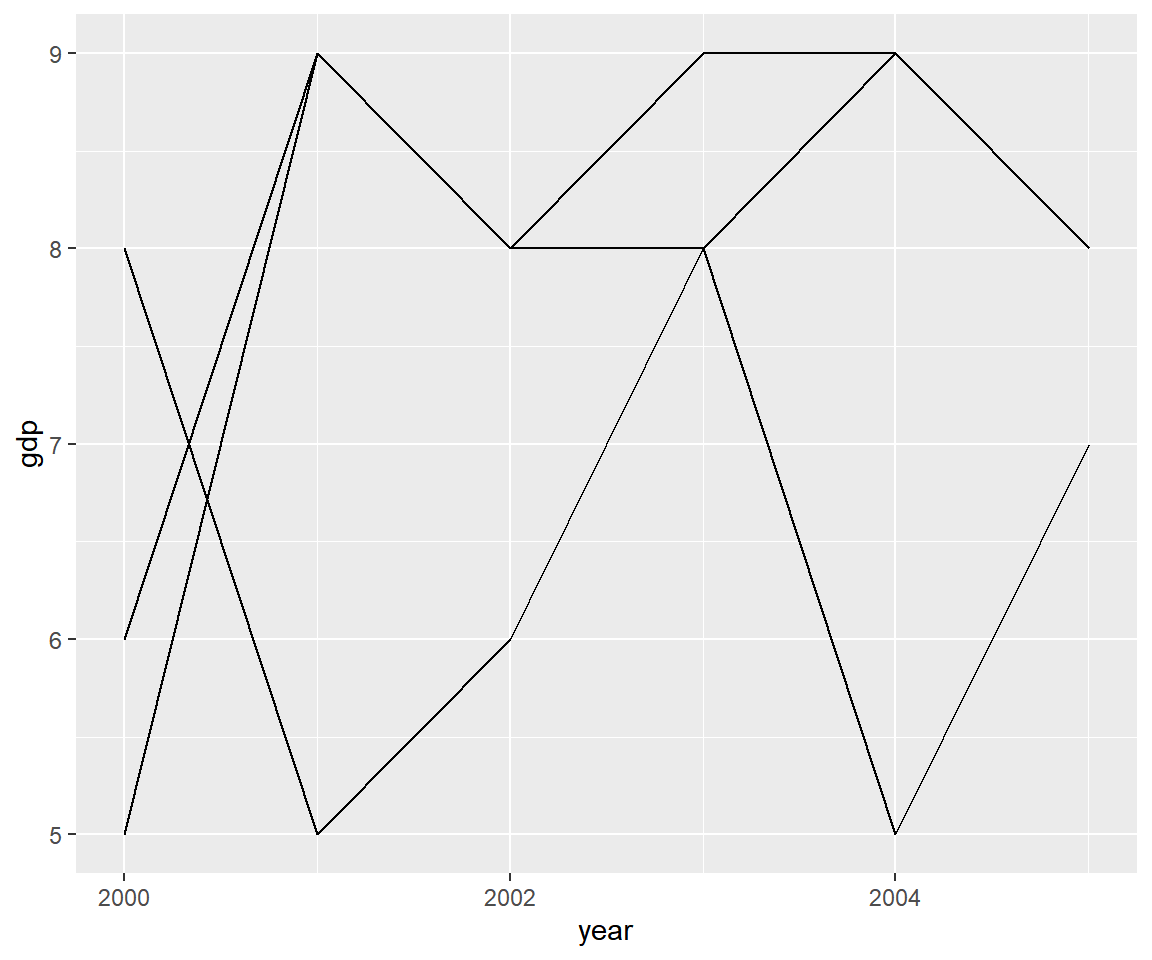Let us begin by ensuring that the lines have different color using the `color` argument within `aes()` and assigning it the variable `country`.

``````ggplot(gdp2, aes(year, gdp, group = country)) +
geom_line(aes(color = country))``````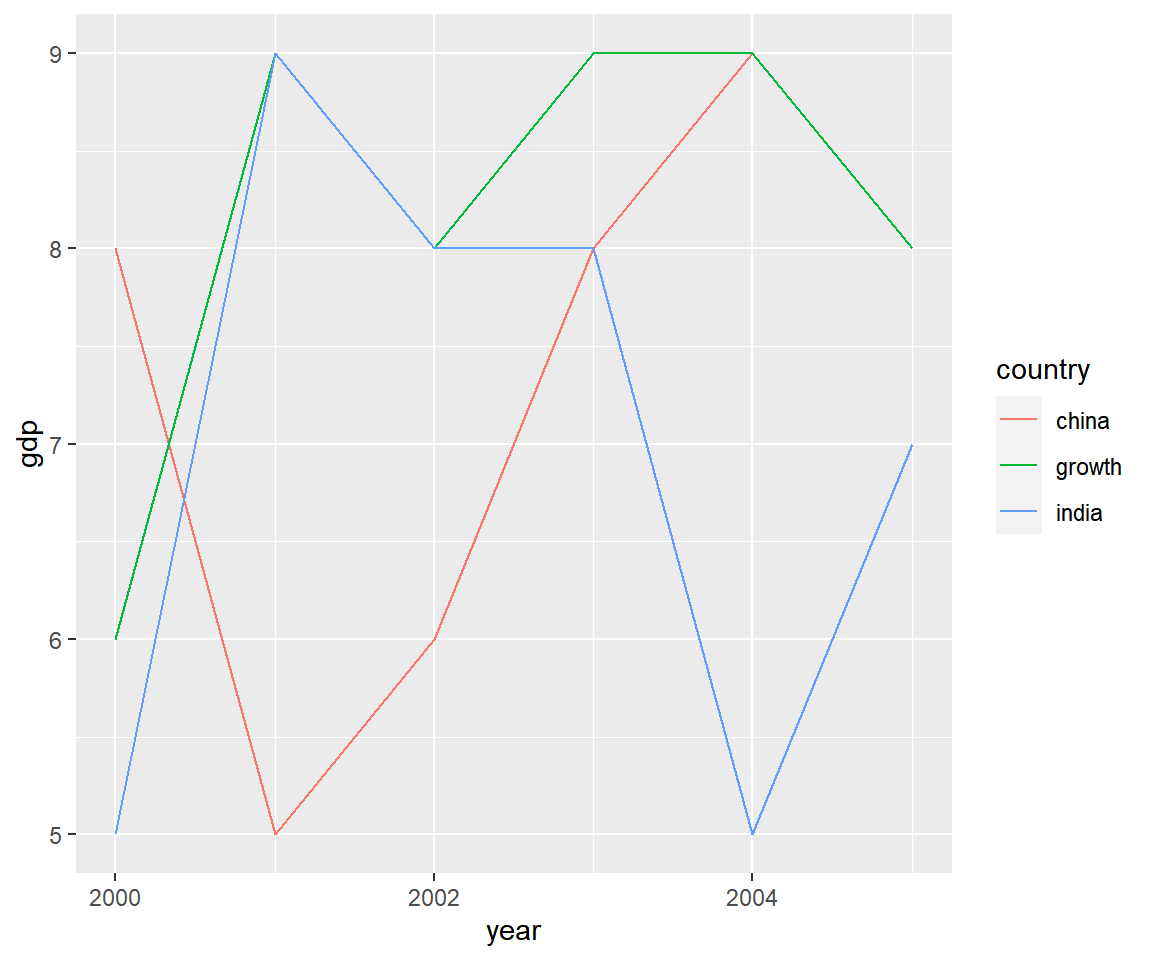Instead of color, now we modify the line type using the `linetype` argument.

``````ggplot(gdp2, aes(year, gdp, group = country)) +
geom_line(aes(linetype = country))``````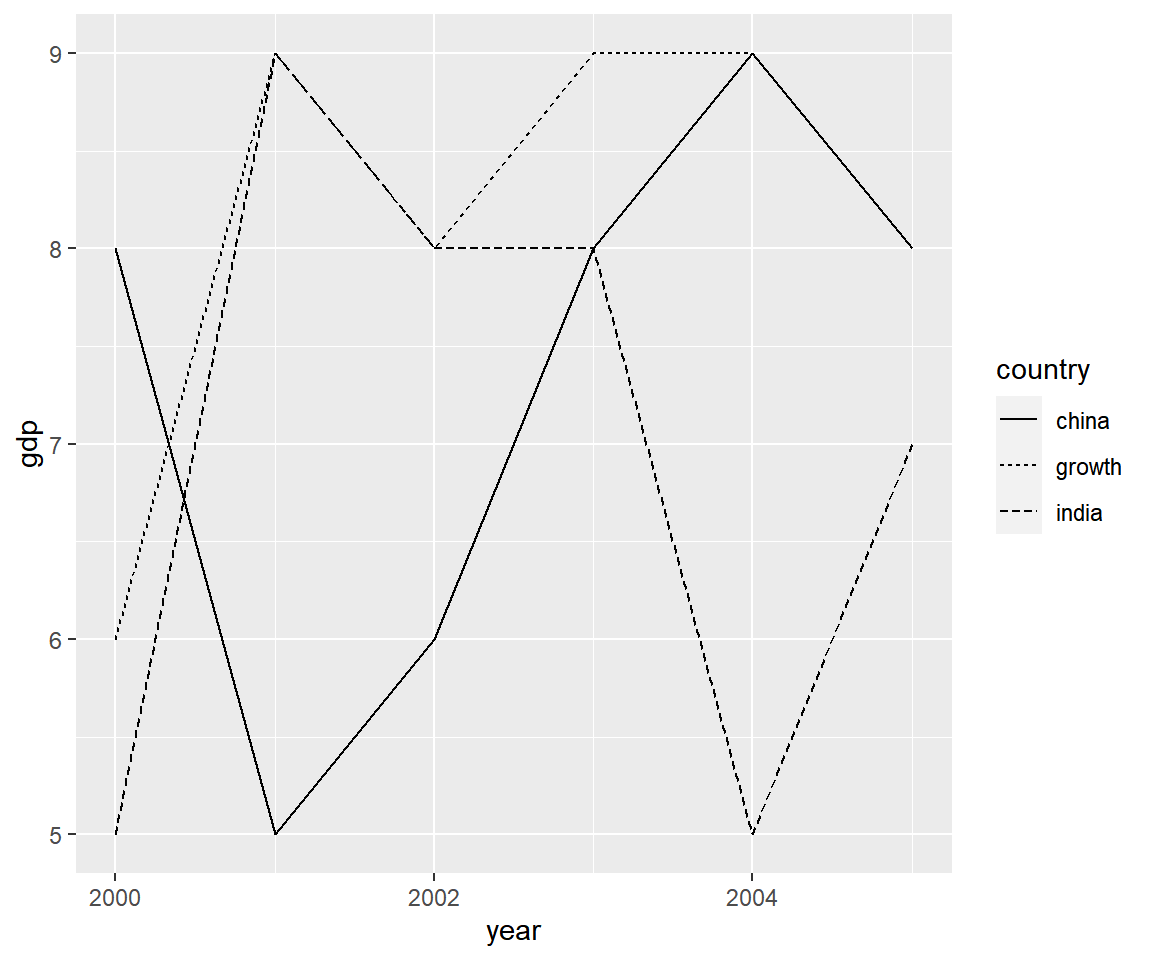In the below instance, we assign different width to the lines using the `size` argument.

``````ggplot(gdp2, aes(year, gdp, group = country)) +
geom_line(aes(size = country))``````
``## Warning: Using size for a discrete variable is not advised.``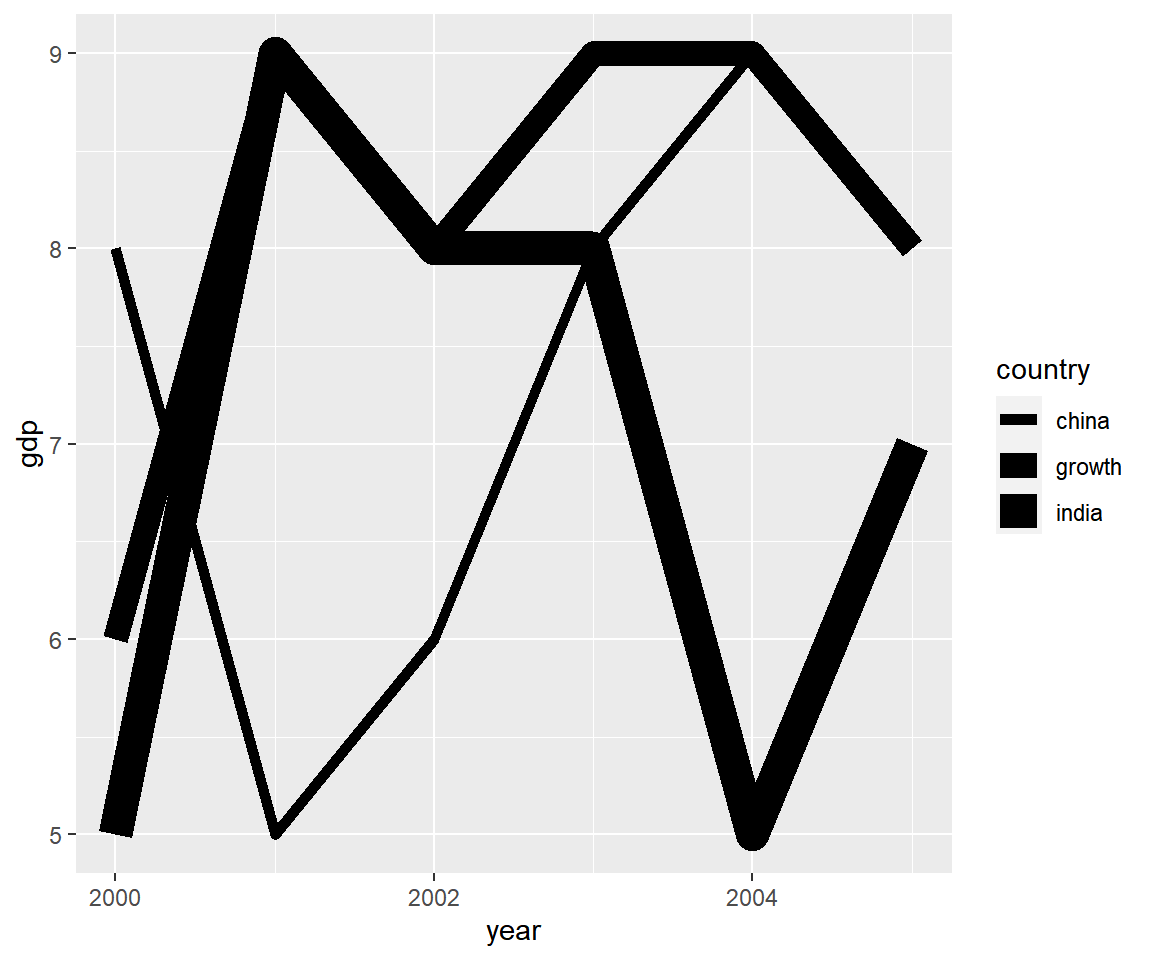Before we wrap up, let us quickly see how we can map aesthetics to variables for different plots.

#### Bar Plots

Here we create a stacked bar plot by mapping `fill` to `purchase`.

``````ggplot(ecom, aes(device, fill = purchase)) +
geom_bar()``````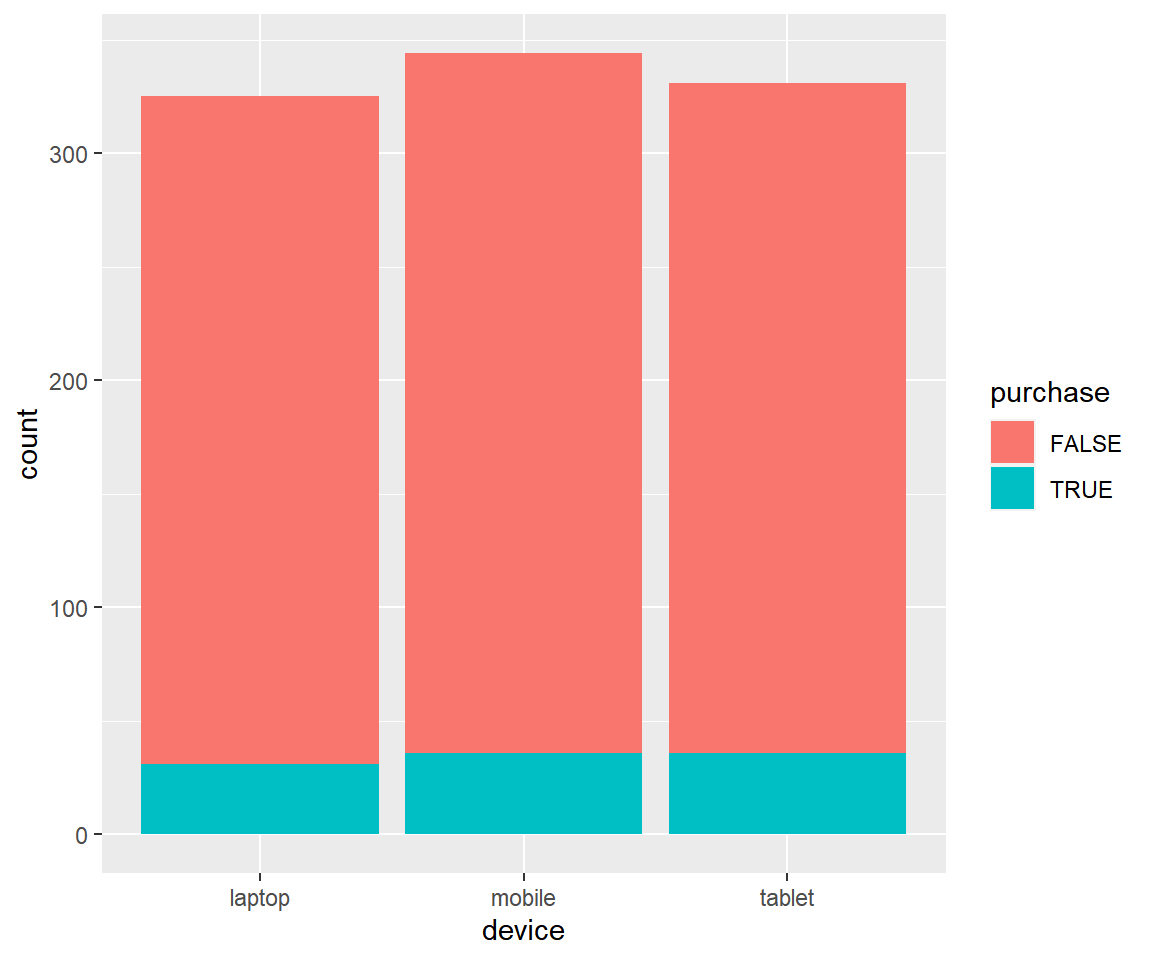#### Histograms

Instead of a bar chart, we create a histogram and again map `fill` to `purchase`.

``````ggplot(ecom) +
geom_histogram(aes(duration, fill = purchase), bins = 10)``````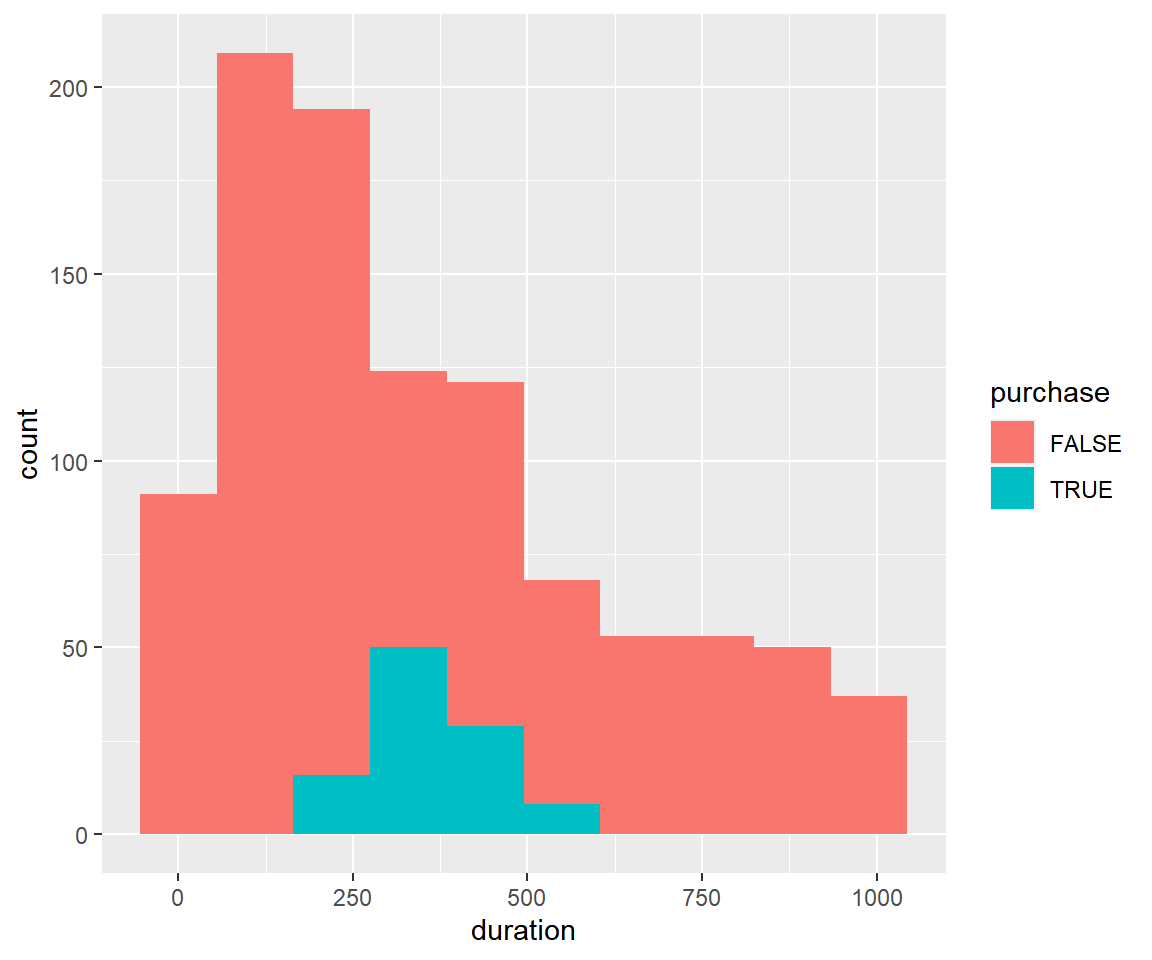#### Box Plots

We repeat the same exercise below, but replace the bar plot with a box plot.

``````ggplot(ecom) +
geom_boxplot(aes(device, duration, fill = purchase))``````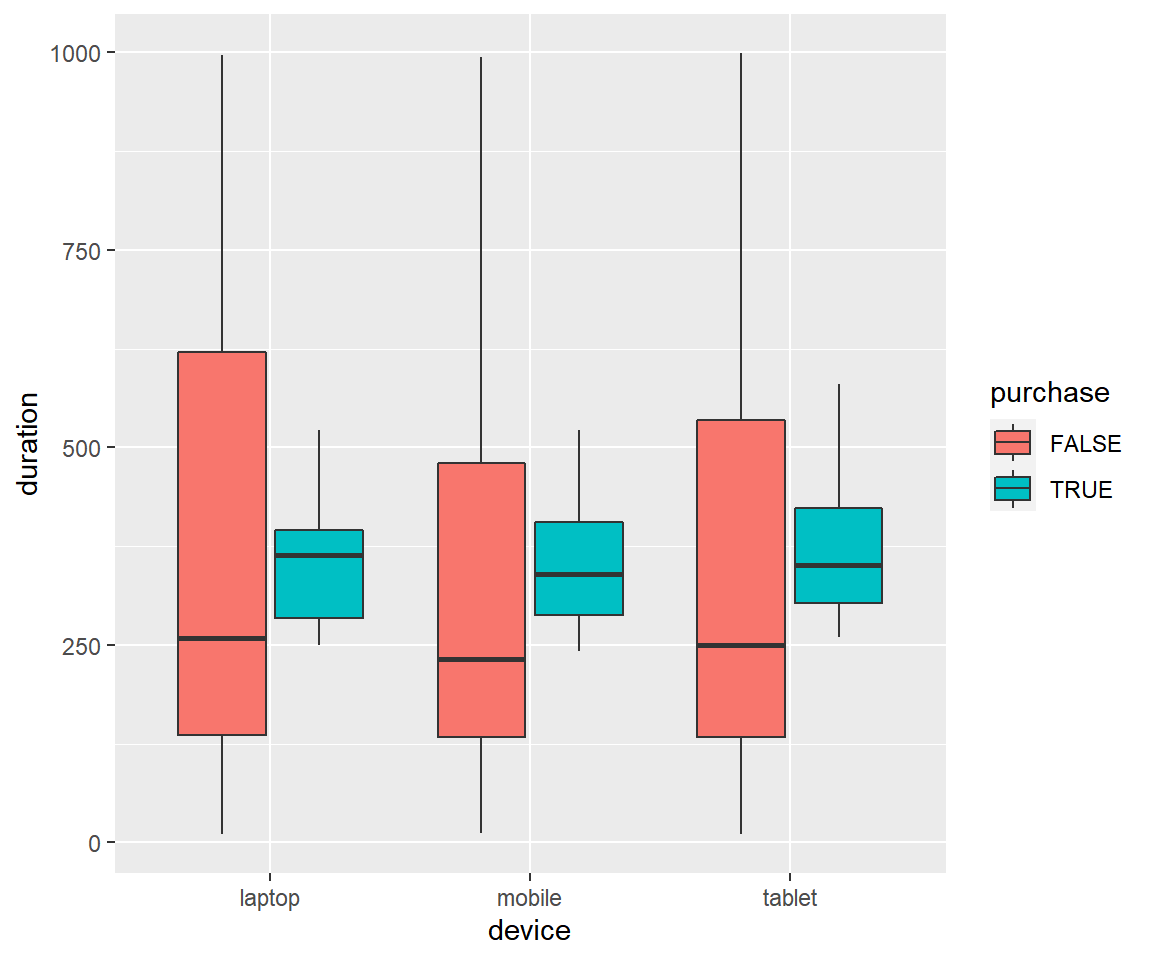In all the above cases, you can observe that when we are mapping aesthetics such as color, fill, shape, size or linetype to variables, they are all wrapped inside `aes()`.

### Summary

In this post, we learnt about aesthetics i.e. how to modify the properties of geoms such as:

• color
• shape
• size
• fill
• alpha
• width

### Up Next..

In the next post, we will learn to modify the axis and labels of a plot.